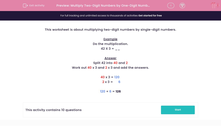# Multiply Two-Digit Numbers by One-Digit Numbers

In this worksheet, students multiply two-digit numbers by one-digit numbers by splitting the larger number if necessary. They use a formal written method.This content is premium and exclusive to EdPlace subscribers.Key stage:  KS 2

Curriculum topic:   Maths and Numerical Reasoning

Curriculum subtopic:   Mixed Problems

Difficulty level:#### Worksheet Overview

This worksheet is about multiplying two-digit numbers by single-digit numbers.

Example

Do the multiplication.

42 X 3 = __

Split 42 into 40 and 2

Work out 40 x 3 and 2 x 3 and add the answers.

40 x 3 = 120

2 x 3 =      6

120 + 6 = 126

### What is EdPlace?

We're your National Curriculum aligned online education content provider helping each child succeed in English, maths and science from year 1 to GCSE. With an EdPlace account you’ll be able to track and measure progress, helping each child achieve their best. We build confidence and attainment by personalising each child’s learning at a level that suits them.

Get started